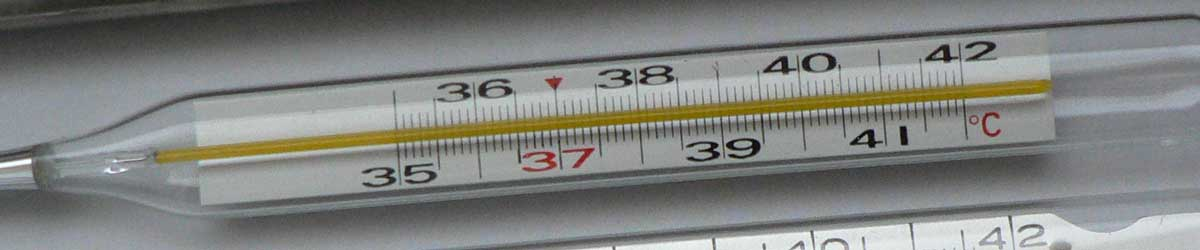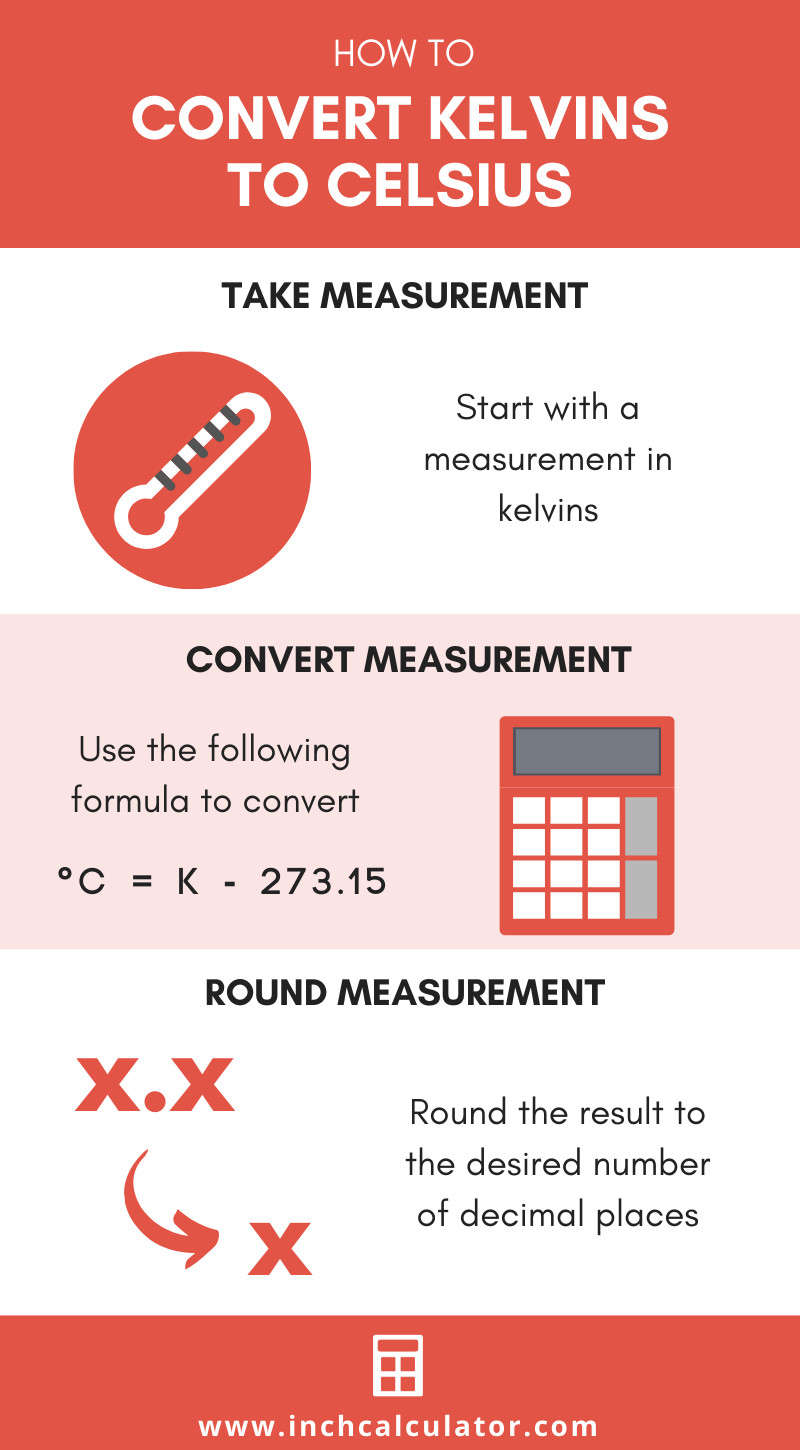# Kelvins to Celsius Conversion

Enter the temperature in kelvins below to get the value converted to Celsius.

Results in Degrees Celsius:1 K = -272.15 °C
Do you want to convert Celsius to kelvins?

## How to Convert Kelvins to CelsiusConvert kelvins to degrees Celsius with this simple formula:

degrees Celsius = [K] - 273.15

The formula to convert kelvins to Celsius is kelvins minus 273.15 Insert the [K] temperature measurement in the formula and then solve to find the result.

For example, let's convert 50 K to degrees Celsius:

50 K = ( 50 - 273.15 ) = -223.15 °C## Kelvins

The Kelvin scale is an absolute temperature scale that uses absolute zero as the starting point. The kelvin is defined in terms of the Boltzmann constant, which is a measure of the energy of motion corresponding to one kelvin.

More specifically, one kelvin is equal to the change of thermodynamic temperature that results in a change of thermal energy kT by 1.380649 × 10−23 J.

0 kelvins are equal to −273.15 degrees Celsius, which is the point of absolute zero.

The kelvin is the SI base unit for temperature in the metric system. Kelvins can be abbreviated as K; for example, 1 kelvin can be written as 1 K.

The kelvin scale is different from other temperature scales in that it is not expressed in degrees, values are expressed in kelvins. When using the Kelvin scale in writing, the "k" is capitalized, however when using the kelvin as a unit the "k" should be lower-case, even though it is named for a person.

### Important Temperatures In Kelvins

TemperatureKelvins
Absolute Zero0 K
Freezing Point of Water273.15 K
Triple Point of Water273.16 K
Boiling Point of Water373.15 K

## Celsius

The Celsius temperature scale, also commonly referred to as the centigrade scale, is defined in relation to the kelvin. Specifically, degrees Celsius is equal to kelvins minus 273.15.

The degree Celsius is the SI derived unit for temperature in the metric system. A degree Celsius is sometimes also referred to as a degree centigrade. Celsius can be abbreviated as C; for example, 1 degree Celsius can be written as 1 °C.

### Important Temperatures In Degrees Celsius

TemperatureDegrees Celsius
Absolute Zero-273.15 °C
Freezing Point of Water0 °C
Triple Point of Water0.01 °C
Boiling Point of Water100 °C

## Kelvin to Celsius Conversion Table

Kelvin measurements converted to Celsius
Kelvins Celsius
0 K -273.15 °C
10 K -263.15 °C
20 K -253.15 °C
30 K -243.15 °C
40 K -233.15 °C
50 K -223.15 °C
60 K -213.15 °C
70 K -203.15 °C
80 K -193.15 °C
90 K -183.15 °C
100 K -173.15 °C
110 K -163.15 °C
120 K -153.15 °C
130 K -143.15 °C
140 K -133.15 °C
150 K -123.15 °C
160 K -113.15 °C
170 K -103.15 °C
180 K -93.15 °C
190 K -83.15 °C
200 K -73.15 °C
273.15 K 0 °C
300 K 26.85 °C
400 K 126.85 °C
500 K 226.85 °C
600 K 326.85 °C
700 K 426.85 °C
800 K 526.85 °C
900 K 626.85 °C
1,000 K 726.85 °C

## References

1. International Bureau of Weights and Measures, The International System of Units, 9th Edition, 2019, https://www.bipm.org/utils/common/pdf/si-brochure/SI-Brochure-9.pdf
2. National Institute of Standards and Technology, SI Units - Temperature, https://www.nist.gov/pml/weights-and-measures/si-units-temperature